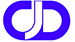Author Message
evren

Joined: 09 Apr 2013
Posts: 2Posted: Wed Mar 16, 2016 10:34 am    Post subject: error 112hello! I' m almost new about plato fortran. I try to write a code about discrete fourier transform. My data is made of ten values(Data is at the bottom of page). When I run it, I get error 112 at the second do loop. Please help me. Thank you for your interest. complex y(0:100),yc dimension x(0:100),amp(0:100),phase(0:100),FRQ(0:100),b(0:100) data pi/3.1415927/ read(*,*) NN N=NN-1 open(3,file='veri.dat',status='old') read(3,2) (x(i),b(i), i=0,NN-1) 2 Format (f5.3,2X,F6.3) write(*,5) (x(i),b(i), i=0,NN-1) 5 Format(F5.3,3X,F6.3) dt=1.0 df=1./nn*dt do 88 k=0,NN (SECOND LOOP BEGINS) do 89 i=0,N yc=cmplx(0,-2*pi*k*df*i) 89 y(k)=y(k)+ b(i)*exp(yc) y(k)=y(k)/NN write(*,*) y(k) 88 CONTINUE DO 30 i=0,N FRQ(i)=i*df amp(i)=cabs(y(i)) phase(i)=atan2(-aimag(y(i)),real(y(i))) write(*,*) amp(i),phase(i) 30 CONTINUE end program time amplitude 0.000 1.000 1.000 2.000 2.000 3.000 3.000 2.000 4.000 0.000 5.000 -0.500 6.000 -1.000 7.000 0.000 8.000 1.000 9.000 0.500[/url][/list][/list]wahorgerJoined: 13 Oct 2014
Posts: 1053
Location: Morrison, CO, USAPosted: Sat Apr 23, 2016 5:14 am    Post subject:The index I runs from 0 to N, but is filled in from 0 to N-1 (aka NN). The b(i) where i=N is undefined.mecej4

Joined: 31 Oct 2006
Posts: 1707Posted: Sat Apr 23, 2016 7:20 am    Post subject:y(k) is not defined when it is used in an expression in the inner DO loop. The array y(:) should perhaps be initialized to zero before the outer loop is started.

Oddly, the array x(:) has data read into it, but it is never used later!

A suggestion to the O.P.: please use the Code button when posting code. Well-indented and syntax-highlighted code is easier to debug for most of us these days.
 Code: program freqp implicit none complex y(0:100),yc real x(0:100),amp(0:100),phase(0:100),FRQ(0:100),b(0:100),pi integer i,n,nn,k real dt,df data pi/3.1415927/ read(*,*) NN N=NN-1 open(3,file='veri.dat',status='old') read(3,2) (x(i),b(i), i=0,N) 2 Format (f5.3,2X,F6.3) write(*,5) (x(i),b(i), i=0,N) 5 Format(F5.3,3X,F6.3) dt=1.0 df=1./nn*dt y(0:NN)=0 do k=0,NN    do i=0,N       yc=cmplx(0,-2*pi*k*df*i)       y(k)=y(k)+ b(i)*exp(yc)    end do    y(k)=y(k)/NN    write(*,*) y(k) end do DO i=0,N    FRQ(i)=i*df    amp(i)=cabs(y(i))    phase(i)=atan2(-aimag(y(i)),real(y(i)))    write(*,*) amp(i),phase(i) end do end program

Last edited by mecej4 on Sat Apr 23, 2016 6:43 pm; edited 2 times in totalwahorgerJoined: 13 Oct 2014
Posts: 1053
Location: Morrison, CO, USAPosted: Sat Apr 23, 2016 2:06 pm    Post subject:Two errors in the same line!! Good catch!Display posts from previous: All Posts1 Day7 Days2 Weeks1 Month3 Months6 Months1 Year Oldest FirstNewest First
 All times are GMT + 1 Hour Page 1 of 1